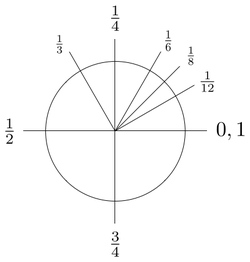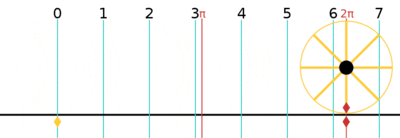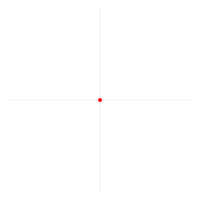# Turn (geometry)

Turn
Unit of Plane angle
Symbol trorpla
Unit conversions
1 tr in ...... is equal to ...
degrees    360°
gons    400gCounterclockwise rotations about the center point where a complete rotation is equal to 1 turn

A turn is a unit of plane angle measurement equal to 2π radians, 360° or 400 gon. A turn is also referred to as a revolution or complete rotation or full circle or cycle or rev or rot.

A turn can be subdivided in many different ways: into half turns, quarter turns, centiturns, milliturns, binary angles, points etc.

## Subdivision of turns

A turn can be divided in 100 centiturns or 1000 milliturns, with each milliturn corresponding to an angle of 0.36°, which can also be written as 21′36″. A protractor divided in centiturns is normally called a percentage protractor.

Binary fractions of a turn are also used. Sailors have traditionally divided a turn into 32 compass points. The binary degree, also known as the binary radian (or brad), is 1256 turn. The binary degree is used in computing so that an angle can be represented to the maximum possible precision in a single byte. Other measures of angle used in computing may be based on dividing one whole turn into 2n equal parts for other values of n.

The notion of turn is commonly used for planar rotations. Two special rotations have acquired appellations of their own: a rotation through 180° is commonly referred to as a half-turn (π radians), a rotation through 90° is referred to as a quarter-turn.

## History

The word turn originates via Latin and French from the Greek word τόρνος (tornos – a lathe).

In 1697, David Gregory used π/ρ (pi over rho) to denote the perimeter of a circle (i.e., the circumference) divided by its radius. However, earlier in 1647, William Oughtred had used δ/π (delta over pi) for the ratio of the diameter to perimeter. The first use of the symbol π on its own with its present meaning (of perimeter divided by diameter) was in 1706 by the Welsh mathematician William Jones. Euler adopted the symbol with that meaning in 1737, leading to its widespread use.

Percentage protractors have existed since 1922, but the terms centiturns and milliturns were introduced much later by Sir Fred Hoyle.

The German standard DIN 1315 (1974-03) proposed the unit symbol pla (from Latin: plenus angulus "full angle") for turns. Since 2011, the HP 39gII and HP Prime support the unit symbol tr for turns. In 2016, support for turns was also added to newRPL for the HP 50g.

## Unit conversionThe circumference of the unit circle (whose radius is one) is 2π.

One turn is equal to 2π (≈ 6.283185307179586) radians.

Conversion of common angles
1/24 turn π/12 rad 15° 16 2/3g
1/12 turn π/6 rad 30° 33 1/3g
1/10 turn π/5 rad 36° 40g
1/8 turn π/4 rad 45° 50g
1/2π turn 1 rad c. 57.3° c. 63.7g
1/6 turn π/3 rad 60° 66 2/3g
1/5 turn 2π/5 rad 72° 80g
1/4 turn π/2 rad 90° 100g
1/3 turn 2π/3 rad 120° 133 1/3g
2/5 turn 4π/5 rad 144° 160g
1/2 turn π rad 180° 200g
3/4 turn 3π/2 rad 270° 300g
1 turn 2π rad 360° 400g

## Tau proposalAn arc of a circle with the same length as the radius of that circle corresponds to an angle of 1 radian. A full circle corresponds to a full turn, or approximately 6.28 radians, which are expressed here using the Greek letter tau (τ).

In 1958, Albert Eagle proposed τ as a symbol for 1/2π, because π resembles two τ symbols conjoined (ττ).

In 2001, Robert Palais proposed using the number of radians in a turn as the fundamental circle constant instead of π, which amounts to the number of radians in half a turn, in order to make mathematics simpler and more intuitive, using a "pi with three legs" symbol to denote the constant (= 2π).

In 2010, Michael Hartl proposed to use the Greek letter tau (τ) instead of pi (π) for two reasons. First, τ is the number of radians in one turn, which allows fractions of a turn to be expressed more directly: for instance, a 3/4 turn would be represented as 3/4τ rad instead of 3/2π rad. Second, τ visually resembles π, whose association with the circle constant is unavoidable. Hartl's Tau Manifesto gives many examples of formulas that are simpler if tau is used instead of pi.

## Examples of use

• As an angular unit, the turn or revolution is particularly useful for large angles, such as in connection with electromagnetic coils and rotating objects. See also winding number.
• The angular speed of rotating machinery, such as automobile engines, is commonly measured in revolutions per minute or RPM.
• Turn is used in complex dynamics for measure of external and internal angles. The sum of external angles of a polygon equals one turn. Angle doubling map is used.
• Pie charts illustrate proportions of a whole as fractions of a turn. Each one percent is shown as an angle of one centiturn.

## Kinematics of turns

In kinematics, a turn is a rotation less than a full revolution. A turn may be represented in a mathematical model that uses expressions of complex numbers or quaternions. In the complex plane every non-zero number has a polar coordinate expression z = r cis(a) = r cos(a) + ri sin(a) where r > 0 and a is in [0, 2π). A turn of the complex plane arises from multiplying z = x + iy by an element u = ebi that lies on the unit circle:

zuz.

Frank Morley consistently referred to elements of the unit circle as turns in the book Inversive Geometry, (1933) which he coauthored with his son Frank Vigor Morley.

The Latin term for turn is versor, which is a quaternion that can be visualized as an arc of a great circle. The product of two versors can be compared to a spherical triangle where two sides add to the third. For the kinematics of rotation in three dimensions, see quaternions and spatial rotation.

## Notes and references

1. "ooPIC Programmer's Guide". www.oopic.com.
2. Hargreaves, Shawn. "Angles, integers, and modulo arithmetic". blogs.msdn.com.
3. "Half Turn, Reflection in Point". cut-the-knot.org.
4. Schwartzman, Steven (1994). The Words of Mathematics: An Etymological Dictionary of Mathematical Terms Used in English. The Mathematical Association of America. p. 165.
5. Croxton, Frederick E. (1922). "A Percentage Protractor". Journal of the American Statistical Association. 18: 108–109. doi:10.1080/01621459.1922.10502455.
6. Hoyle, Fred (1962). Astronomy. London: Macdonald.
7. German, Sigmar; Drath, Peter (2013-03-13) . Handbuch SI-Einheiten: Definition, Realisierung, Bewahrung und Weitergabe der SI-Einheiten, Grundlagen der Präzisionsmeßtechnik (in German) (1 ed.). Friedrich Vieweg & Sohn Verlagsgesellschaft mbH, reprint: Springer-Verlag. ISBN 3322836061. 978-3-528-08441-7, 9783322836069. Retrieved 2015-08-14.
8. Kurzweil, Peter (2013-03-09) . Das Vieweg Einheiten-Lexikon: Formeln und Begriffe aus Physik, Chemie und Technik (in German) (1 ed.). Vieweg, reprint: Springer-Verlag. doi:10.1007/978-3-322-92920-4. ISBN 3322929205. 978-3-322-92921-1. Retrieved 2015-08-14.